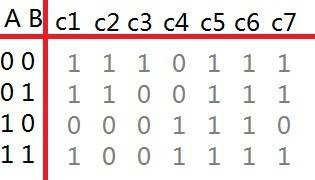# 7 segment display + logic gates

QuarkCharmer

## Homework Statement

I'm tasked with creating a logic network that can spell out a simple 4 letter word. It has 2 inputs A and B, and 7 outputs (for a 7 segment display). The 2 bits (A and B) give the 4 total possible inputs to the circuit.

Write the truth table for the 7 output functions, minimize them using a K-map, and implement them using logic gates.

## The Attempt at a Solution

I've created the truth table.But I don't really understand how I am supposed to use K-maps to simplify this.
Am I supposed to create 7 functions, for example:
C1 = A'B' + A'B +AB
C2 = A'B' + A'B
C3 = A'B'
... and so on, up to the seventh.

Then use the Kmap to minimize those functions?